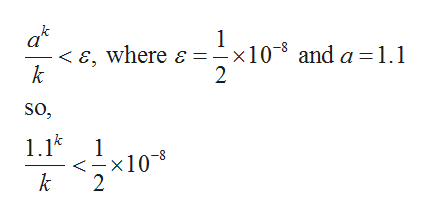# Determine how many terms are needed to compute:ln 1.1 with error less than (1/2)×10^(−8) using alternating series theorem and Taylor series of ln(1 + x) about x = 0.

Question
54 views

Determine how many terms are needed to compute:
ln 1.1 with error less than (1/2)×10^(−8) using alternating series theorem and Taylor series of ln(1 + x) about x = 0.

check_circle

Step 1

From the given information it is needed to calculate the number of terms for ln(1.1) with error less than 1/2×10-8 by using the alternative series theorem and taylor series expansion of ln(1+x) about x=0.

So, use the form of remainder in taylor series. The lagrange form for the remainder on the closed interval from a to x is:

Step 2

Now, it is required to calculate the value of k such that the remainder Rk(1.1)

for the kth order  taylor series for ln(x) about x=0 is less than 1/2×10-8

Step 3

So, to calculate the valu...help_outlineImage Transcriptionclose1 < E, where e =-x10 2 and a 1.1 k so 1.1 -x10 k 1 2 fullscreen

### Want to see the full answer?

See Solution

#### Want to see this answer and more?

Solutions are written by subject experts who are available 24/7. Questions are typically answered within 1 hour.*

See Solution
*Response times may vary by subject and question.
Tagged in

### Math